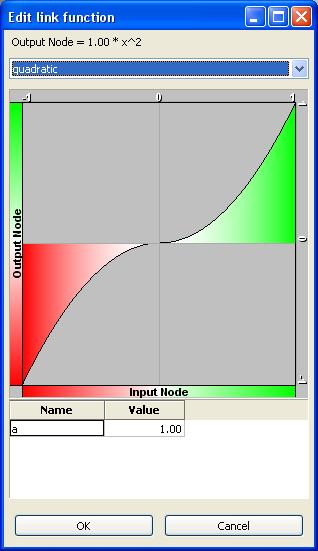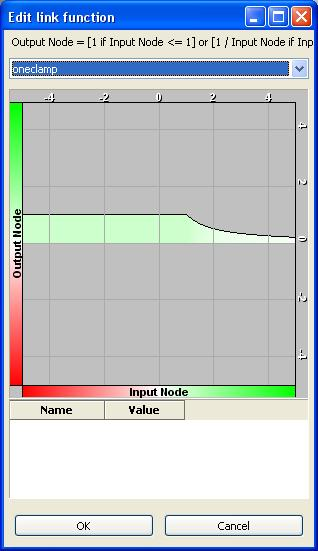# FaceFX Support

FaceFX Documentation and support

Link functions connect two nodes in the face graph. Values start at the input node and flow through the link function to the output node. The input node’s value can be thought of as x, and the link function can be thought of as some function of x: f(x).

The graph you see when you modify a link function demonstrates the range of possible values that link function can input and output. The X-axis is determined by the input node’s min and max values, while the y-axis is determined by the output node’s min and max values. The link function’s graph represents the function’s traversal of this 2-dimensional space. By default, red indicates negative values while green indicates positive values.

### Linear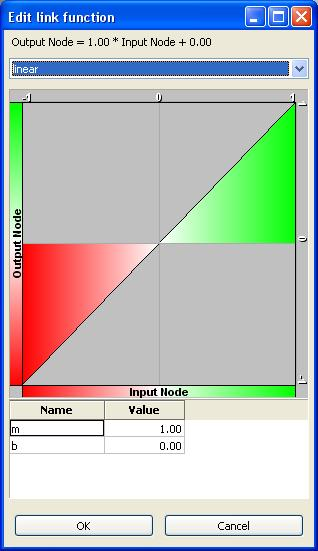Most functions you will create in the face graph will be linear with b=0. As you drive up one node’s value, the other node’s value changes at a rate depending on the slope described by the variable m.

#### Variables

m - The slope of the linear link function b  - The Y-intercept of the linear function

### Clamped Linear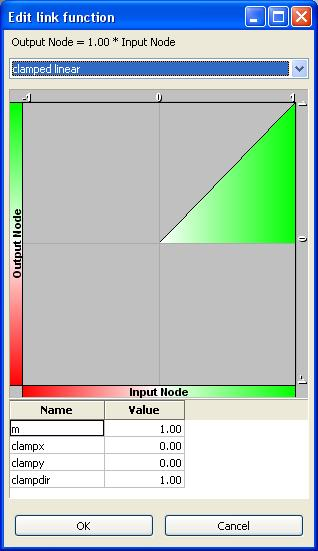The clamped linear function joins a linear link function with a constant link function. Depending on the value of x, the output value is determined by either the linear link function or the constant. Clamped Linear functions are used in the sample content’s gaze direction setup.

#### Variables

m - The slope of the linear link function clampx - The x-value where the linear link function becomes constant. clampy - The y-value where the linear link function becomes constant. clampdir - At the point (clampx, clampy), the clamped linear function switches between a linear link function and a constant link function. The clampdir variable determines which function is evaluate to the right of the point and which is evaluated to the left. Clampdir values greater than zero indicate that the linear link function should be evaluated to the right of the point and constant link functions should be evaluated to the left. Values for clampdir equal to or less than zero do the opposite.

### Constant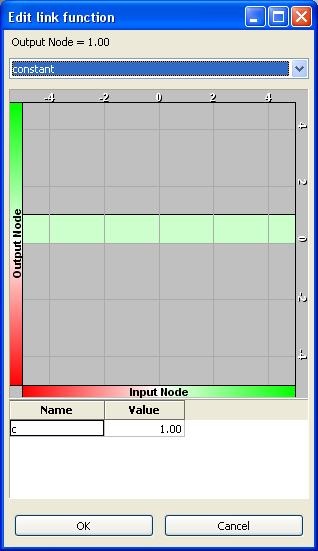Constant link functions do not depend on the input node’s value. They always output the same value regardless of the input. As a result, it is irrelevant what node is the input node.

### Corrective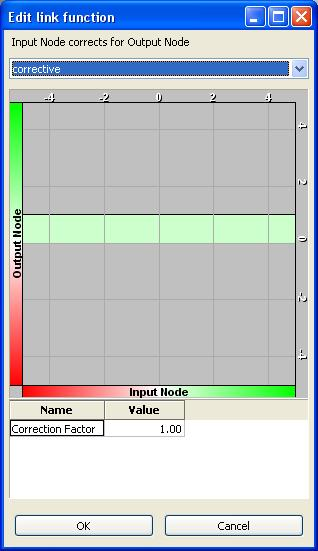When two targets A and B conflict with each other but target A is more important, you can use a corrective link function from A to B. When A and B are both active, A forces B’s value down. The amount of correction is determined by the “Correction Factor”

###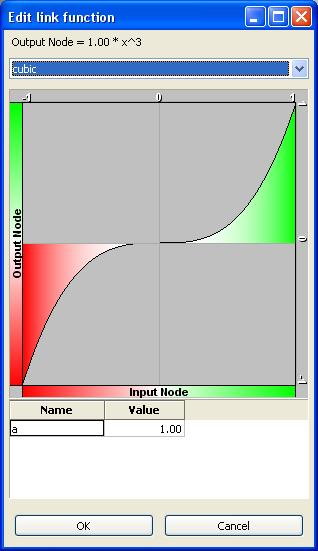A cubic link function.

### Inverse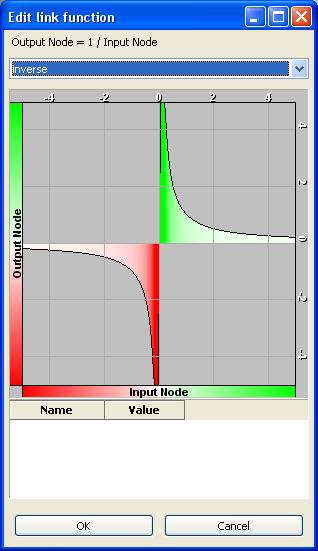In the Face Graph, the inverse function’s output is 0 when x = 0.

### Negate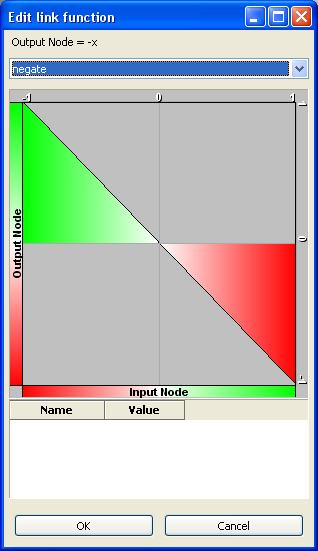Negate is simply a linear link function with m = -1 and b=0.

### Square Root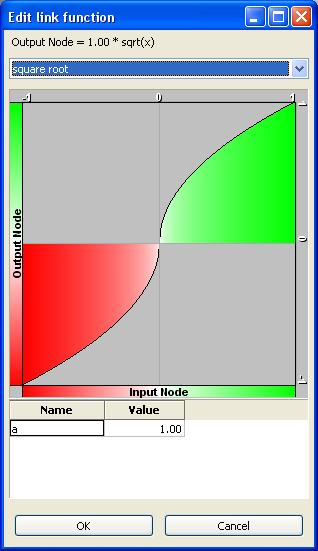Warning:  Square root is slow!